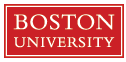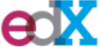Feedback

Save course to Your Buffet - Get notified, Track Progress, Plan Future Learning.
8 People Have this course in their Buffet

#### Introduction to Differential Equations

Classified as: MATH230 - Differential Equations (1 Alt. Course Available)
Course Description:

Phenomena as diverse as the motion of the planets, the spread of a disease, and the oscillations of a suspension bridge are governed by differential equations. MATH226x is an introduction to the mathematical theory of ordinary differential equations. This course follows a modern dynamical systems approach to the subject. In particular, equations are analyzed using qualitative, numerical, and if possible, symbolic techniques. MATH226x is essentially the edX equivalent of MA226, a one-semester course in ordinary differential equations taken by more than 500 students per year at Boston University. It is divided into three parts. MATH226.1x is the first of these three parts. In MATH226.1x, we will discuss biological and physical models that can be expressed as differential equations with one or two dependent variables. We will discuss geometric/qualitative and numerical techniques that apply to all differential equations. When possible, we will study some of the standard symbolic solution techniques such as separation of variables and the use of integrating factors. We will also study the theory of existence and uniqueness of solutions, the phase line and bifurcations for first-order autonomous systems, and the phase plane for two-dimensional autonomous systems. The techniques that we develop will be used to analyze models throughout the course.

• Instructor(s) Paul Blanchard, Patrick Cummings
• University• Provider• Start Date 29/Jan/2015
• Duration 7 weeks
• Main Language English
Did you find any errors in this course listing ? Help us improve and we would be eternally grateful

#### Course Reviews

• No Comments Yet! Be the first one to comment.